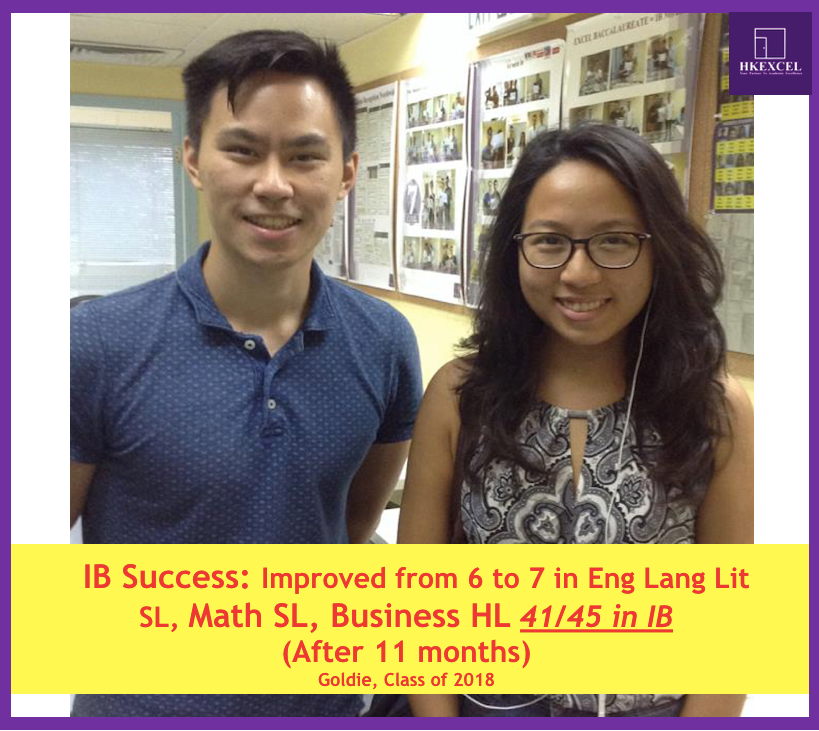top of page
Search

# How can an IB Math tutor dramatically boost your IB scores and what is the exam structure of IB math

Math is one of the most important subjects in IB, some top tier schools even specifically ask for a 7 in math. So that’s why many IB students are looking for the right IB Math tutor in order to boost their IB math scores.

HKExcel has helped over 1000 math students since 5 years ago and was able to boost grade for students. Our IB math tutor even helped a student who used to get predicted a level 1 at school in math SL , but he finally got boosted up to a level 6 in his final grade in IB, which is truly unbelievable to his peers and teachers, as those used to mock him and said he is destined to fail in math. But he proved them wrong.

Exam structure

Knowing the exam structure of IB math paper is extremely vital to your success. IB math has Math studies SL , Math SL and Math HL. They have different setting in terms of IB exam paper.

For math studies, you will only have two paper, which is paper 1 & 2 and they are all Calculator papers, that means you do not need to calculate math by hand in math studies. Paper 1 is short questions, consists of 15 questions, full mark is 90. So each question counts 6 marks around while paper 2 is long questions , you will see 6 questions in the paper, and each questions is around 15 marks, divided into 4 to 5 parts, like (a) , (b) , (c), (d) etc. So what you need to know in math studies is that , you need to know your calculator well and also don’t try to calculate the math by hand to avoid careless mistakes, as you can always use your calculator to do the math.

For Math SL, you will also be examined by two papers, however the setting is different from math studies, papers full mark is still 90. The paper has 2 sections, A & B , and A consists of 7 short questions around, which count 45 marks, while B has 3 long questions , each count 15 marks. The difference between math studies and math sl ,Apart from combined short question and long questions in one paper, its Paper 1 is non-calculator paper, which is a lot harder and test more on students’ ability to process math by hand, which is more challenging. So in math sl, you need to have a better time management , especially in paper 1 , but you do not need to be afraid that in the non-calculator paper, you will be asked to solve complicated numbers with multiple digits or decimals, the IB department has quite a humane setting for paper 1 in which the number will usually be integers for easy calculator, the main goal of paper 1 is to test out your ability to solve questions and understandings of a certain topics.

Lastly , for Math HL , which is hardest level in IB math. You will have 3 papers. And for paper 1 and 2 , the setting is exactly same as math sl, which is short question and long question combined, but of course the questions will be more challenging and harder. In paper 3, it is what we called the option paper, you can choose 1 out of 4 options, which are Sets, Statistics, Calculus and Discrete math. Some schools do not allow you to choose some of the options, since those topics are extremely advanced. Most interestingly, some school teachers may not have learnt all the options before, so that’s why they will force to choose those they have learnt, and if you insist to do other options, then you might need to self study. However, hkexcel math department has developed all option notes and we have sorted all chapter past exam questions and also have our own concept notes, so students can easily absorb the concept well and also practice with the exact IB questions. With this training, we believe students will not only be able to gain confidence in math , but also learn the correct way to ace IB math exam.

Our IB math tutor team is committed to making IB easier for you and improving your results. Click here to register for a FREE trial if you are interested to boost your IB Math score!